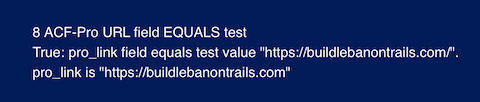# Test for values in shortcodes for Advanced Custom Fields

You can test the field connection shortcodes for the following Advanced Custom Fields (third-party plugin) with these operators:

• Text, Email, or Url-type fields
Test if the value equals or doesn't equal a text string you specify.
• Number field
Test if the value equals, doesn't equal, is greater than, greater than or equal to, less than, less than or equal to an integer you specify.
• Radio Button field (as of Beaver Themer 1.2.1)
Test for the value of the radio button selected. See the Related Articles for ACF Radio Button examples in various types of field connections.

Warning: When you use a value test, make sure the fields you're evaluating have a value. If the field is empty, the value test will always return FALSE. For example, if you're testing whether the field equals the string "abc" and the field is empty, an EQUALS test returns FALSE, and a NOTEQUALS test also returns FALSE. The best way to ensure the field has a value is to make it a required field.

The syntax is the same as for the standard Themer Boolean-If and the if-else conditional shortcode statements (see the Related Articles below), with extra parameters `exp` and `value`.

In the following example (and the other examples in the following section), the custom field is identified with the standard `post:acf` (to identify the field as an ACF), `type` (the ACF field type, such as `text`, `number`, `radio`), and `name` (your custom field name), then the `exp` option for the operator and the field `value` being tested.

```[wpbb-if post:acf type='text' name='auto-make'
exp='equals' value='Porsche']
This text displays when the value test is true.
[wpbb-else]This text displays when the value test is false.
[/wpbb-if]
```

For Text, Email, and Url fields, you can use the following operator values for the `exp` option.

• `equals`
• `notequals`

For the Number field, you can use the following operator values for the `exp` option.

• `equals`
• `notequals`
• `greater`
• `greaterequals`
• `less`
• `lessequals`

The `value` parameter is the value that you want to test for.

Notes:

• When the value of `exp` is `greater`, `greaterequals`, `less`, or `lessequals`, both the Number field value and the value you're testing for in the value parameter are converted to integers before they're compared, but `equals` and `notequals` compare the exact strings that are there. So, for example, if you're testing whether 377.78 is LESSEQUALS 377.77, the result is TRUE, because both values are converted to 377 before comparison occurs, so they are equal. On the other hand, if you test whether 377.77 EQUALS 377.78, the result is FALSE. because the two strings are being compared as is.
• The EQUALS test for URLs ignores the trailing slash if there is one, in both the field value and the comparison value. For example, https://example.com and https://example.com/ will return TRUE to the EQUALS test.
• The `value` parameter is case-sensitive.

## Examples of testing ACF

The following examples of conditional shortcode statements were inserted into an HTML module in a Singular Themer layout. They were constructed so the shortcode value would be displayed, as well as whether the test for the value is true or false. The following custom fields were created for these tests using the Advanced Custom Fields plugin.

• `string_value` (type Text)
• `number_value` (type Number)
• `email_address` (type Email)
• `pro_link` (type Url, a type only available in the Pro version)

Look at the output in each example to help understand the code.

#### 1 ACF Text field EQUALS example

```1 Text field equals test<br>
[wpbb-if post:acf type='text' name='string_value' exp='equals' value='try me out']
True: ACF field called string_value equals the test value "try me out".<br>
string_value is "[wpbb post:acf type='text' name='string_value']"
[wpbb-else]False: ACF field called string_value does not equal the test value "try me out".<br>
string_value is "[wpbb post:acf type='text' name='string_value']"
[/wpbb-if]
```

Output: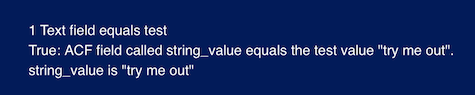#### 2 ACF Text field NOTEQUALS example

```2 Text field notequals test<br>
[wpbb-if post:acf type='text' name='string_value' value='try' exp='notequals']
True: The ACF field string_value does not equal the test value "try".<br>
string_value is "[wpbb post:acf type='text' name='string_value']"
[wpbb-else]  False: The ACF field string_value does not equal the test value "try"<br>
string_value is "[wpbb post:acf type='text' name='string_value']"
[/wpbb-if]
```

Output: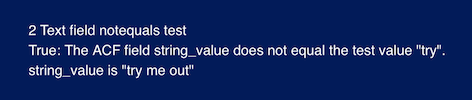#### 3 ACF Number field LESS example

```3 ACF Number field less test<br>
[wpbb-if post:acf type='number' name='number_value' exp='less' value='37809']
True: The ACF number_value field is less than 37809.<br>
The number field value is [wpbb post:acf type='number' name='number_value']
[wpbb-else]False: The ACF number_value field isn't less than 37809. .<br>
The number field value is [wpbb post:acf type='number' name='number_value']
[/wpbb-if]
```

Output: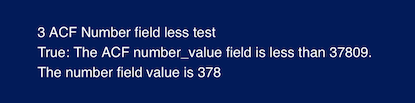#### 4 ACF Number field GREATER example

```4 ACF Number field greater test<br>
[wpbb-if post:acf type='number' name='number_value' exp='greater' value='378']
True: The number field value is greater than 378.<br>
The number field value is [wpbb post:acf type='number' name='number_value'].
[wpbb-else] False: The number field value isn't greater than 378.<br>
The number field value is [wpbb post:acf type='number' name='number_value'].
[/wpbb-if]
```

Output: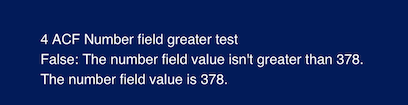#### 5 ACF Number field GREATEREQUALS example

```5 ACF Number field greaterequals test<br>
[wpbb-if post:acf type='number' name='number_value' exp='greaterequals' value='378']
True: The number field value is greater than or equals 378.<br>
The number field value is [wpbb post:acf type='number' name='number_value'].
[wpbb-else] False: The number field value isn't greater than or equals 378.<br>
The number field value is [wpbb post:acf type='number' name='number_value'].
[/wpbb-if]
```

Output: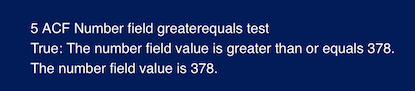#### 6 ACF Number field NOTEQUALS example

Remember the EQUALS and NOTEQUALS comparisons don't convert number values into integers first, so the comparison is for an exact match of two strings.

```6 ACF Number field notequals test<br>
[wpbb-if post:acf type='number' name='number_value' exp='notequals' value='377']
True: The number field value does not equal 377.<br>
The number field value is [wpbb post:acf type='number' name='number_value'].
[wpbb-else] False: The number field value equals 377.<br>
The number field value is [wpbb post:acf type='number' name='number_value'].
[/wpbb-if]
```

Output: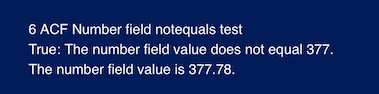#### 7 ACF Email field EQUALS test

Remember that if a field is empty, both the EQUALS test and NOTEQUALS test return FALSE.

```7 ACF Email field equals test<br>
[wpbb-if post:acf type='email' name='email_address' exp='equals' value="fred@example.com"]
True: ACF field email_address equals test value "fred@example.com".<br>
[wpbb-else]False: ACF field email_address doesn't equal test value "fred@example.com".<br>
[/wpbb-if]
```

Output: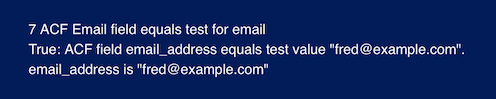#### 8 ACF-Pro URL EQUALS example

Remember that the trailing slash is ignored in both the field and the comparison values.

```8 ACF-Pro URL field EQUALS test<br>
[wpbb-if post:acf type='url' name='pro_link' exp='equals' value='https://buildlebanontrails.com/']
True: pro_link field equals test value "https://buildlebanontrails.com/".<br>